# 14 Results

View
Selected filters:
• GeometryConditional Remix & Share Permitted
CC BY-NC-SA
Rating

This textbook is an introductory coverage of algorithms and data structures with application to graphics and geometry.

Subject:
Mathematics
Geometry
Material Type:
Textbook
Provider:
Global Text Project
Author:
Jurg Nievergelt
Klaus Hinrichs
01/01/2011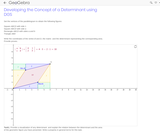Conditional Remix & Share Permitted
CC BY-SA
Rating

Developing the Concept of a Determinant using DGS

Subject:
Algebra
Geometry
Material Type:
Simulation
Provider:
GeoGebra
Provider Set:
GeoGebraTube
11/11/2011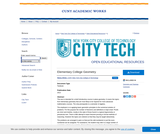Conditional Remix & Share Permitted
CC BY-NC-SA
Rating

This text is intended for a brief introductory course in plane geometry. It covers the topics from elementary geometry that are most likely to be required for more advanced mathematics courses. The only prerequisite is a semester of algebra.

The emphasis is on applying basic geometric principles to the numerical solution of problems. For this purpose the number of theorems and definitions is kept small. Proofs are short and intuitive, mostly in the style of those found in a typical trigonometry or precalculus text. There is little attempt to teach theorem-proving or formal methods of reasoning. However the topics are ordered so that they may be taught deductively.

The problems are arranged in pairs so that just the odd-numbered or just the even-numbered can be assigned. For assistance, the student may refer to a large number of completely worked-out examples. Most problems are presented in diagram form so that the difficulty of translating words into pictures is avoided. Many problems require the solution of algebraic equations in a geometric context. These are included to reinforce the student's algebraic and numerical skills, A few of the exercises involve the application of geometry to simple practical problems. These serve primarily to convince the student that what he or she is studying is useful. Historical notes are added where appropriate to give the student a greater appreciation of the subject.

This book is suitable for a course of about 45 semester hours. A shorter course may be devised by skipping proofs, avoiding the more complicated problems and omitting less crucial topics.

Subject:
Geometry
Material Type:
Textbook
Provider:
Provider Set:
New York City College of Technology
Author:
Henry Africk
01/01/2013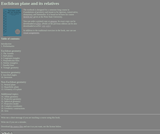Conditional Remix & Share Permitted
CC BY-SA
Rating

This book is designed for a semester-long course in Foundations of Geometry and meant to be rigorous, conservative, elementary and minimalistic.

Subject:
Geometry
Material Type:
Textbook
Author:
Anton Petrunin
08/13/2018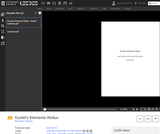Conditional Remix & Share Permitted
CC BY-NC
Rating

"Euclid's 'Elements' Redux" is an open textbook on mathematical logic and geometry based on Euclid's "Elements" for use in grades 7-12 and in undergraduate college courses on proof writing.

Many problem solvers throughout history wrestled with Euclid as part of their early education including Copernicus, Kepler, Galileo, Sir Isaac Newton, Ada Lovelace, Abraham Lincoln, Bertrand Russell, and Albert Einstein. This edition is part of an effort to ensure that tomorrow's great thinkers will have that same privilege.

Subject:
Geometry
Material Type:
Textbook
Author:
Daniel Callahan
10/29/2021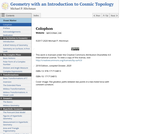Conditional Remix & Share Permitted
CC BY-SA
Rating

Motivated by questions in cosmology, the open-content text Geometry with an Introduction to Cosmic Topology uses Mobius transformations to develop hyperbolic, elliptic, and Euclidean geometry - three possibilities for the global geometry of the universe.

The text, written for students who have taken vector calculus, also explores the interplay between the shape of a space and the type of geometry it admits. Geometry is suitable for a semester course in non-Euclidean geometry or as a guide to independent study, with over 200 exercises and several essays on topics including the history of geometry, parallax and curvature, and research aimed at determining the shape of the universe.

Subject:
Geometry
Material Type:
Textbook
Author:
Michael P. Hitchman
04/27/2020Conditional Remix & Share Permitted
CC BY-NC
Rating

Law of CosinesTMM 002 PRECALCULUS (Revised March 21, 2017)2c. Analyze general triangles. Routine analysis of side lengths and angle measurements using trigonometric ratios/functions, as well as other relationships.*Sample Tasks:The student can solve general triangles using trigonometric ratios and relationships including laws of sine and cosine.The student can compare similar triangles.The student can compute length and angle measurements inside complex drawings involving multiple geometric objects.The student can algebraically describe relationships inside complex drawings involving multiple geometric objects.

Subject:
Mathematics
Calculus
Geometry
Trigonometry
Material Type:
Module
Provider:
Ohio Open Ed Collaborative
05/28/2019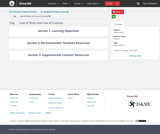Conditional Remix & Share Permitted
CC BY-NC
Rating

Law of SinesTMM 002 PRECALCULUS (Revised March 21, 2017)2c. Analyze general triangles. Routine analysis of side lengths and angle measurements using trigonometric ratios/functions, as well as other relationships.*Sample Tasks:The student can solve general triangles using trigonometric ratios and relationships including laws of sine and cosine.The student can compare similar triangles.The student can compute length and angle measurements inside complex drawings involving multiple geometric objects.The student can algebraically describe relationships inside complex drawings involving multiple geometric objects.

Subject:
Higher Education
Mathematics
Calculus
Geometry
Trigonometry
Material Type:
Module
Provider:
Ohio Open Ed Collaborative
05/28/2019Conditional Remix & Share Permitted
CC BY-NC
Rating

Vectors - dot product, projection, decomposition of a vectorTMM 002 PRECALCULUS (Revised March 21, 2017)AdditionalOptional Learning Outcomes:2. Geometry: The successful Precalculus student can:2e. Interpret the result of vector computations geometrically and within the confines of a particular applied context (e.g., forces).Sample Tasks:The student can define vectors, their arithmetic, their representation, and interpretations.The student can decompose vectors into normal and parallel components.The student can interpret the result of a vector computation as a change in location in the plane or as the net force acting on an object.

Subject:
Engineering
Higher Education
Mathematics
Geometry
Trigonometry
Material Type:
Module
Provider:
Ohio Open Ed Collaborative
05/28/2019Conditional Remix & Share Permitted
CC BY-NC
Rating

Vectors - magnitude, direction, component form, trigonometric form, unit vector, algebra of vectors, applications,TMM 002 PRECALCULUS (Revised March 21, 2017)AdditionalOptional Learning Outcomes:2. Geometry: The successful Precalculus student can:2e. Interpret the result of vector computations geometrically and within the confines of a particular applied context (e.g., forces).Sample Tasks:The student can define vectors, their arithmetic, their representation, and interpretations.The student can decompose vectors into normal and parallel components.The student can interpret the result of a vector computation as a change in location in the plane or as the net force acting on an object.

Subject:
Higher Education
Mathematics
Calculus
Geometry
Physics
Material Type:
Module
Provider:
Ohio Open Ed Collaborative
05/28/2019Conditional Remix & Share Permitted
CC BY-NC
Rating

Ellipse - conic section, foci, major and minor axes, vertices, standard form, eccentricityTMM 002 PRECALCULUS (Revised March 21, 2017)AdditionalOptional Learning Outcomes:2. Geometry: The successful Precalculus student can:2f. Represent conic sections algebraically via equations of two variables and graphically by drawing curves.Sample Tasks:The student can perform the process “completing the square” transforming the equation into a standard form.The student can draw curves representing conic sections.The student can solve systems of equations involving linear and quadratic functions.The student can parametrize conic curves.

Subject:
Higher Education
Mathematics
Algebra
Geometry
Astronomy
Material Type:
Module
Provider:
Ohio Open Ed Collaborative
05/28/2019Conditional Remix & Share Permitted
CC BY-NC
Rating

Hyperbola - conic section, foci, transverse and conjugate axes, vertices, asymptotes, standard formTMM 002 PRECALCULUS (Revised March 21, 2017)AdditionalOptional Learning Outcomes:2. Geometry: The successful Precalculus student can:2f. Represent conic sections algebraically via equations of two variables and graphically by drawing curves.Sample Tasks:The student can perform the process “completing the square” transforming the equation into a standard form.The student can draw curves representing conic sections.The student can solve systems of equations involving linear and quadratic functions.The student can parametrize conic curves.

Subject:
Higher Education
Mathematics
Geometry
Astronomy
Material Type:
Module
Provider:
Ohio Open Ed Collaborative
05/28/2019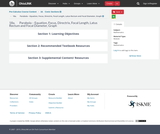Conditional Remix & Share Permitted
CC BY-NC
Rating

Parabola - conic section, focus, directrix, focal length, standard form, vertex form, completing the square method.TMM 002 PRECALCULUS (Revised March 21, 2017)AdditionalOptional Learning Outcomes:2. Geometry: The successful Precalculus student can:2f. Represent conic sections algebraically via equations of two variables and graphically by drawing curves.Sample Tasks:The student can perform the process “completing the square” transforming the equation into a standard form.The student can draw curves representing conic sections.The student can solve systems of equations involving linear and quadratic functions.The student can parametrize conic curves.

Subject:
Higher Education
Mathematics
Algebra
Geometry
Material Type:
Module
Provider:
Ohio Open Ed Collaborative
05/28/2019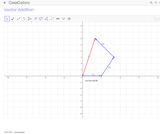Conditional Remix & Share Permitted
CC BY-SA
Rating

Addition of three Vectors, and displays resultant Vectors. Can drag the endPoints of the three different Vectors, but the resultant always starts at the origin.

Subject:
Geometry
Material Type:
Simulation
Provider:
GeoGebra
Provider Set:
GeoGebraTube